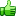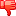Might be useful to someone: Line of sight testing in a 2D tile based game
Posted by Billy
Posted on 28 May, 2014 at 4:56PM10

The following C++ function does line-of-sight testing for a 2D game that uses tiles. The level map is a multidimensional array of integers (levelmap[LEVEL_HEIGHT][LEVEL_WIDTH]) that represent each tile; 0 signifies and empty tile, any number higher than that is solid. A vector2 is just a datatype that contains an object's x and y position.

// Check line of sight between two points (usually the camera/player)
// Based on Bresenham's line algorithm
// Returns true if line of sight is achieved
bool CheckLine(sf::Int8 levelmap[LEVEL_HEIGHT][LEVEL_WIDTH], sf::Vector2f A, float rotationA, sf::Vector2f B) const
{
// Make sure A is facing towards B

// Get angle we'd need to look straight at B
float angle = (float)(-atan2( (double)B.x - A.x , (double)B.y - A.y) * 180 / M_PI - 180);
// Keep rotation in a nice 360 circle
if(angle <= -1) angle = 360 + angle;
if(angle >= 360) angle = angle - 360;
// Now check if our rotation falls in our field of view, 60 degrees on each side
if(rotationA < angle - 60 || rotationA > angle + 60)
return false;

// Now, check the line of sight
bool steep = (fabs(B.y - A.y) > fabs(B.x - A.x));
if(steep)
{
std::swap(A.x, A.y);
std::swap(B.x, B.y);
}

if(A.x > B.x)
{
std::swap(A.x, B.x);
std::swap(A.y, B.y);
}

float dx = B.x - A.x;
float dy = fabs(B.y - A.y);

float error = dx / 2.0f;
int yStep = (A.y < B.y) ? 1 : -1;
int y = (int)A.y;

int maxX = (int)B.x;

int x;
for(x=(int)A.x; x < maxX; x++)
{
if(steep)
{
if(levelmap[x / TILE_WIDTH][y / TILE_HEIGHT] != 0) return false;
}
else
{
if(levelmap[y / TILE_HEIGHT][x / TILE_WIDTH] != 0) return false;
}

error -= dy;
if(error < 0)
{
y += yStep;
error += dx;
}
}

return true;
}28 May, 2014 at 5:44PM00